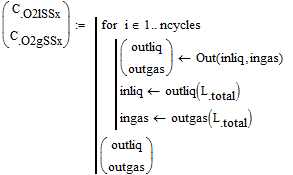cancel
Showing results for
Did you mean:
cancel
Showing results for
Did you mean:

SOLVED

## Re: Help for iteration

Fred, in case you can't open and view the OPs worksheet, here is a pdf print of it.

He had already solved the ODE system and I have no clue which iteration he is asking for.

## Re: Help for iteration

Hey Werner & Fred, What i meant by iteration is a for loop.

I have a system with a fix inlet and outlet which has a length of 250 m.

1 cycle means that the system run from inlet into outlet for 1 time.

When i run the derivation, i use intial value of Clin (concentration liquid in) and Cgin (concentration gas in) which will give a result of Clout (concentration liquid out) and Cgout (concentration gas out).

For the 2nd cycle, i want to use Clout for the initial value instead of Clin. And for the gas, i will always want to use Cgin as intial value (fresh air).

Clin1cycle = Clin & Clin2cycle = Clout1cycle & Clin3cycle = Clout2cycle, etc.

Cgin1cycle = Cgin2cycle = Cgin2cycle = Cgin

However, i dont know how to translate in into for loop command in mathcad.

That's what i was trying to ask.

Thank you

## Re: Help for iteration

Is the attached what you are looking for?

## Re: Help for iteration

Is the attached what you are looking for?

Alan

## Re: Help for iteration

Hey Alan,

I think yes but i do not understand the outliq (L.total) and outgas(L.total) term and do i have to add inside the ODE solver the determination of cycles ?## Re: Help for iteration

@NandaV wrote:

Hey Alan,

I think yes but i do not understand the outliq (L.total) and outgas(L.total) term and do i have to add inside the ODE solver the determination of cycles ?The number of cycles is set by the variable ncycles that is assigned immediately before the program structure. I've arbitrarily set ncycles to 5.  You will need to set it to whatever is appropriate for your problem.

I've assumed that for given inlet values of gas and liquid, the calculations are done for every section (x) from 1 to Ltotal. Within the program I've used dummy variables outliq and outgas to calculate  the values of CO2l and CO2g for every section (x) (via the Odesolve block, which is now a function of the inlet values).  The inlets for the next cycle, inliq and ingas  are then set to the outlet values of outliq and outgas for the previous cycle, hence they are set to the last section values, namely outliq(Ltotal) and inliq(Ltotal). The final values of outliq and outgas each a vector of length Ltotal, are then returned to CO2lSS and CO2gSS respectively.

Alan

Edit:  My description above is not completely correct!  outliq and outgas are not vectors, but functions (of x), so that CO2lSS and CO2gSS are also functions.

Alan

(For some reason my previous reply was posted twice!!)

## Re: Help for iteration

@Werner_E wrote:

Fred, in case you can't open and view the OPs worksheet, here is a pdf print of it.

He had already solved the ODE system and I have no clue which iteration he is asking for.

Thanks Werner,

Smath will open parts of xmcd drawings (not Prime), but it just skips what it doesn't understand (things like "Find" and graphs.  So I can speak to some of the problems, but not what I can't see. . .

## Re: Help for iteration

Ah.. okay I understand. But is it possible to do the derivation only once?

I mean now I have the for loop cycle after the 1st cycle derivation.

Also, isn't now the system taking the value from previous x point, so the COlin (x) = COlout (X-1) instead of per cycle? (I hope you understand what I meant).

## Re: Help for iteration

@NandaV wrote:

I mean now I have the for loop cycle after the 1st cycle derivation.

No. I just left an example of a single cycle so you could see that changing the Odesolve block to a function didn't alter the calculation.  In the attached I've removed this!

@NandaV wrote:

Also, isn't now the system taking the value from previous x point, so the COlin (x) = COlout (X-1) instead of per cycle?

No. Each cycle covers all values of x before updating the inlet values for the next cycle.  This is because all values of x are considered by the Odesolve function which is called just once per cycle.

Alan

## Re: Help for iteration

Yes. I understand now. Thank you so much Alan!